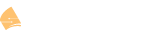# EIGRP Wide Metrics

This topic is to discuss the following lesson:

Hi Rene,
I have a question. What is the difference between composite metric and vector metric in EIGRP?
I see both the metric when I issue the command “sh ip eigrp x.x.x.x”

I appreciate if you can visualize me the difference between them.

Regards,
Mallik

Hello Mallik,

Although I am not Rene, I hope I can help you out. From what I understand vector metrics are the metrics that in total make up the composite metric. For example Bandwidth, Delay, Load, Reliability, and MTU are the Vector Metrics. Together they are used in a formula to find the composite metric. This composite metric is used by EIGRP to decide the best paths to insert into the topology and routing table.

Below is a quote and link to a Cisco article that you might find helpful.

"EIGRP Composite Cost Metrics
The Enhanced Interior Gateway Routing Protocol (EIGRP) uses bandwidth, delay, reliability, load, and K values (various constants that can be configured by a user to produce varying routing behaviors) to calculate the composite cost metric for local Routing Information Base (RIB) installation and route selections. The EIGRP composite cost metric is calculated using the following formula:

EIGRP composite cost metric = 256*((K1Scaled Bw) + (K2Scaled Bw)/(256 – Load) + (K3Scaled Delay)(K5/(Reliability + K4)))

EIGRP uses one or more vector metrics to calculate the composite cost metric. The table below lists EIGRP vector metrics and their descriptions"

I hope this helps you understand EIGRP better!

Thanks,
Scott

2 Likes

Hello Scott

That’s absolutely correct. Each of the K values (K1, K2, K3, K4, K5) are vectors and the composite metric is the result of the stated formula.

Thanks!

Laz

Many thanks Scott, well explained…appreciate your help.

2 Likes

Good day Network Lessons Team!
I was investigating the formula of calculating short composite metric with the relic components from IGRP: Load, along with the Reliability.
A)
256*((K1Scaled Bandwidth) + (K2Scaled Bandwidth)/(256 – Load) + (K3Scaled Delay)(K5/(Reliability + K4)))

I saw a lot of sources where we can find the same formula A , but there is another one B (the last parameter (K5/(Reliability + K4) is out of parentheses)
B)
256 * [K1Scaled Bandwidth + K2Scaled Bandwidth/(256 – Load) + K3*Scaled Delay] * K5/(Reliability + K4)

They give us different results. I tested it in GNS3 and observe that formula B is right. What have I missed?

Hello Evgeny

Looking at the RFC that describes EIGRP, the formula indicated is indeed formula “A” as stated in your post. Can you share with us where you found formula “B”? Also, it is true that you will indeed get different results with the different formulas, but this is the case only if you take K4 and K5 into account. Can you share your specific configuration with us that you performed in GNS3 to help us in responding?

I look forward to hearing from you.

Laz

Hello! Nice to hear you!

Formula B
CCIE R&S v5.0 vol.1 fifth edition
Narbik and Peter are writers, page 364

Formula A

Any EIGRP configuration in GNS3 at Cisco 7200, 3745 with “metric weights 0 1 0 1 1 1” gives me metrics of routes, and their values show me that formula B was used.

Hello again Evgeny

I found the reference in the Cert guide and started reading, and I realized that the two formulas are actually the same. This is because, for multiplication, the order of operations doesn’t matter. For example, if you have

256 * (a+b+c) * d

this is exactly the same as

256 * [(a+b+c) * d]

which is also exactly the same as

(256a + 256b + 256c) * d

You can replace

• a with (K1Scaled Bandwidth)
• b with (K2Scaled Bandwidth)/(256 – Load)
• c with (K3Scaled Delay)
• d with (K5/(Reliability + K4))

and you have the same thing. So it seems that both formulas will indeed bring the same result, because they are actually the same. I think the number of brackets has made it more difficult to see.

I hope this has been helpful!

Laz

Lazaros,
if my eyes don’t let me down
the formula A from the topic is:
256 * (a + b + c * d)

the formula B from the book is:
256 * (a + b + c) * d

Hello Evgeny

You’re right, the equation in the Cisco documentation you linked to is indeed formula A as shown below:

I turns out that this equation is wrong. This is a typo. (I didn’t look at this specific equation closely enough before, sorry…)

The equation from the RFC 7868 which is shown below is correct, and agrees with the equation in the Cert Guide:

``````5.6.1.1.  Classic Composite Formulation

EIGRP calculates the composite metric with the following formula:

metric = 256 * ({(K1*BW) + [(K2*BW)/(256-LOAD)] + (K3*DELAY)} * (K5/(REL+K4)))
``````

So to finallyanswer your question correctly, the correct equation is B, and the specific Cisco documentation has a typo.

I hope this has been helpful!

Laz

Thank you for clarifying!

1 Like